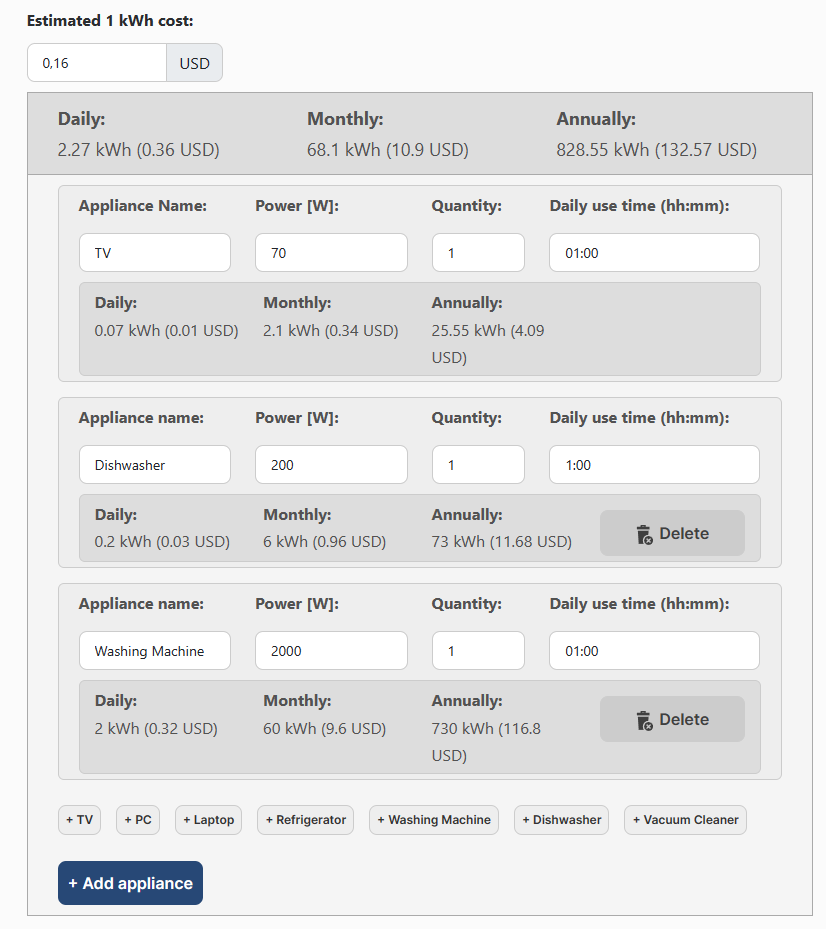# Energy Consumption Calculator

Calculate estimated electricity consumption and cost based on the power consumption of your devices, and their daily operating time.

USD
Daily:
0 kWh (0 USD)
Monthly:
0 kWh (0 USD)
Annually:
0 kWh (0 USD)
Daily:
0 kWh (0 USD)
Monthly:
0 kWh (0 USD)
Annually:
0 kWh (0 USD)

The Energy Consumption Calculator helps you determine how much energy an appliance uses based on its power and average daily usage time. The calculator displays the daily, monthly, and yearly energy usage of each appliance and the total energy consumption of all appliances entered.

## How to use this Calculator?

1. If you want to calculate the approximate costs, enter the estimated cost per kilowatt-hour (kWh).
2. Add the first appliance by specifying its name, power consumption in watts (W), quantity (e.g. if you have multiple devices), and daily usage time in hours and minutes (hh:mm).
4. View the results. The daily, monthly, and yearly energy consumption of each appliance is displayed below it. At the top of the calculator, you'll find a summarized result for all appliances entered.

For example:As you can see, there are results displayed below each appliance, but they are also summarized at the top of the calculator for all appliances entered.

## How to calculate daily Energy Consumption?

Multiply the power (W) by the daily operating time (hours/day), and divide the result by 1000.

Energy [kWh/day] = Power [W] × Time [h/day] / 1000

For instance, let's calculate the daily energy consumption (in kWh) of a TV that has a power consumption of 70 watts and is used for an average of 3 hours per day.

Energy [kWh/day] = 70 × 3 / 1000 = 0.21 kWh

## Examples

Appliance name Power Daily use time Daily Monthly Annually
Laptop 60 W 03:00 0.18 kWh 5.4 kWh 65.7 kWh
Dishwasher 200 W 01:00 0.2 kWh 6 kWh 73 kWh
Refrigerator 200 W 24:00 4.8 kWh 144 kWh 1752 kWh
Financial Calculators
PureTables.com
Have an idea for a tool or calculator that would make your life easier? Contact us, so we could try to create and provide it.